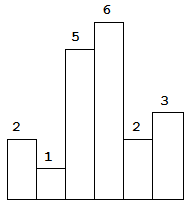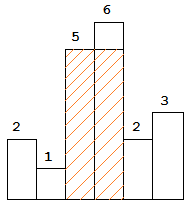# LeetCode_084: Largest Rectangle in Histogram

经典的题目，最开始没想到这种解法，只想着用优先队列去解了，看了下答案，大概有两种解法，一种是DP，另一种是维护一个栈，这种解法应该是见过最好的了。惯例，贡献高质量解法。

### 题目

Given n non-negative integers representing the histogram’s bar height where the width of each bar is 1, find the area of largest rectangle in the histogram.Above is a histogram where width of each bar is 1, given height = [2,1,5,6,2,3].The largest rectangle is shown in the shaded area, which has area = 10 unit.

For example,
Given heights = [2,1,5,6,2,3],
return 10.

### 分析

For example,
Given height = [2,1,5,6,2,3],
return 10.

1、如果已知height数组是升序的，应该怎么做？

2、使用栈的目的就是构造这样的升序序列，按照以上方法求解。

（1）2进栈。s={2}, result = 0

（2）1比2小，不满足升序条件，因此将2弹出，并记录当前结果为2*1=2。

（3）5比1大，满足升序条件，进栈。s={1,5},result = 2

（4）6比5大，满足升序条件，进栈。s={1,5,6},result = 2

（5）2比6小，不满足升序条件，因此将6弹出，并记录当前结果为6*1=6。s={1,5},result = 6

2比5小，不满足升序条件，因此将5弹出，并记录当前结果为5*2=10（因为已经弹出的5,6是升序的）。s={1},result = 10

2比1大，将弹出的5,6替换为2重新进栈。s={1,1,2,2,2},result = 10  注：这里s事实上只存储了1,2的下表，可以恢复它们的位置，这里为了方便理解，就写出来直观的意思了。

（6）3比2大，满足升序条件，进栈。s={1,1,2,2,2,3},result = 10

在代码中，与上面的描述有两点不同，一是栈s存储的是数组下标的索引，二是在直方图末尾增加一个0作为哨兵，方便计算最后一个bar引起的面积（0可以使所有元素出栈，也就是上面第六步中的最后计算）。

### 代码

class Solution {
public:
int largestRectangleArea(vector<int>& heights) {
int res = 0;
stack<int> si;
heights.push_back(0); // NIL
const int n = heights.size();
for (int i = 0; i < n; ++i){
while (!si.empty() && heights[si.top()] >= heights[i]){
int h = heights[si.top()];
si.pop();
int s = h * (si.empty() ? i : (i - si.top() - 1));
res = max(res, s);
}
si.push(i);
}
return res;
}
};